peteroznewman
Subscriber

Hello Drbn,

My test case consists of three tubes modeled using beams that come together at a common point where a load is applied that puts two of the beams into compression and one of the beams into tension. At three support points, each beam has a zero displacement and the axial rotation is zeroed. A remote point on one beam is used to apply a force.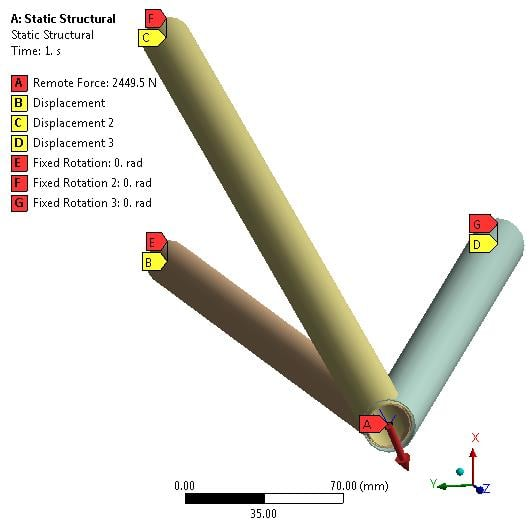The remote point is scoped to one beam and two spherical joints connect that remote point to the other two beams.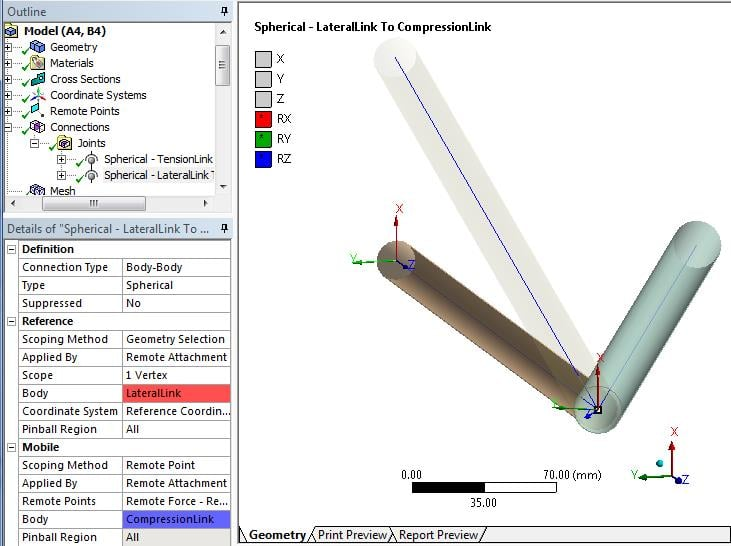In DesignModeler, three Parameters are created for the OD of each tube.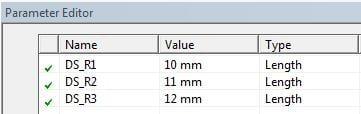The wall thickness of all tubes is set to 0.5 mm by subtracting that value off the OD to define the ID.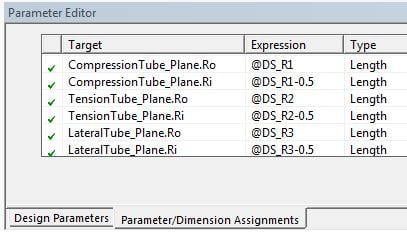The Workbench Project schematic includes a Buckling analysis and a Direct Optimization.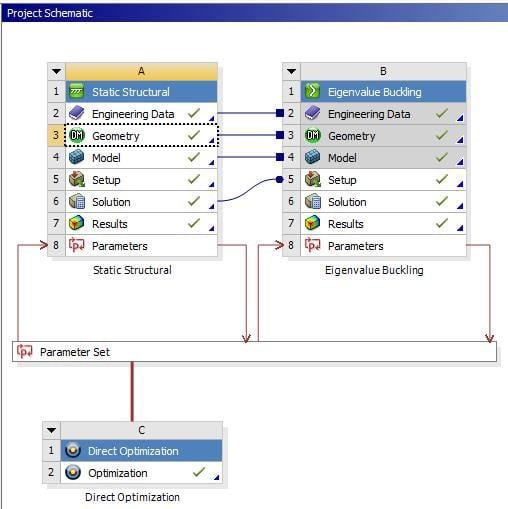The Parameter Set shows two Outputs selected from the Static Structural and one from the Eigenvalue Buckling solution.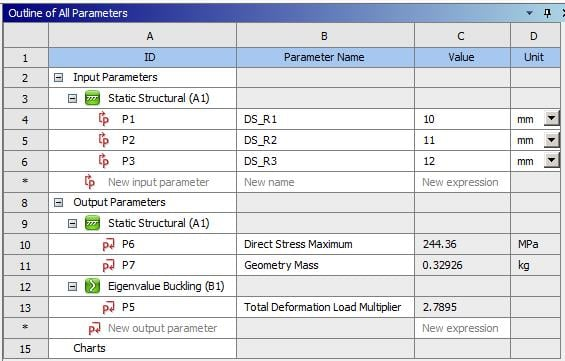The input parameter upper and lower bounds are shown below.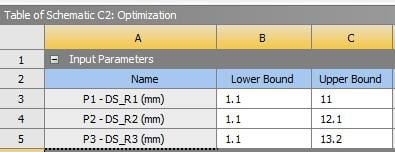Optimization was defined as shown below: minimize mass, keeping Load Multiplier > 1 and Stress < 250 MPa.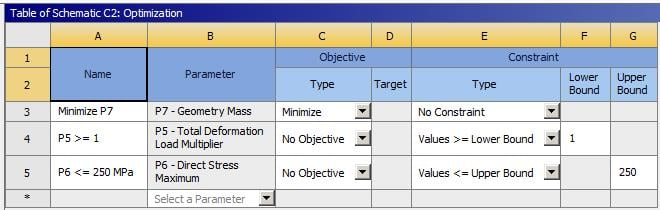The Direct Stress Maximum was to keep the tension member from exceeding the yield strength.
I could have added a Direct Stress Minimum to keep the compression members from exceeding yield strength.

The buckling Load Multiplier applies to both beams. So if R1< R3 the first beam will buckle.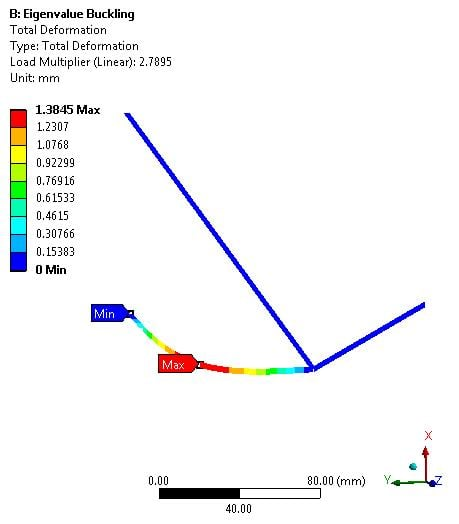But if R1 > R3, the second beam will buckle.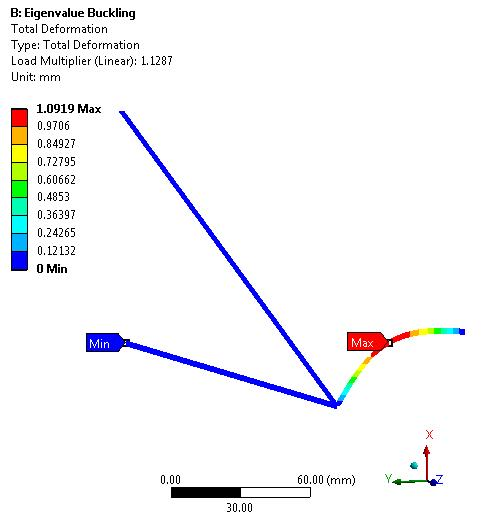The scoping for the buckling Load Multiplier is to All Bodies, so the constraint keeps the beam most likely to buckle from having a Load Mulitplier < 1.

The Direct Optimizer solved the model 48 times to come up with an optimum solution.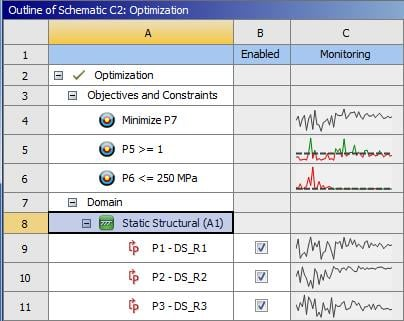I hope you can apply this to your research.

Attached is the ANSYS 19.1 archive.

Regards,

Peter